# Periodic Motion, Waves & Sound Flashcards Preview

## AP Physics > Periodic Motion, Waves & Sound > Flashcards

Flashcards in Periodic Motion, Waves & Sound Deck (97):
1

## Where is the amplitude of the waveform shown below?### Amplitude is the distance between the average value of the waveform and the extreme value of the waveform.Don't fall into the trap of thinking the amplitude is the full range from minimum to maximum; that is actually twice the amplitude.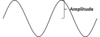2

## What is the amplitude of the waveform below, if each vertical division represents 1 cm?### The amplitude of the wave is 3 cm. Don't make the mistake of thinking the amplitude covers the entire wave, from minimum to maximum; that value (6 cm in this case) is twice the actual amplitude.3

## What is the period of the waveform shown below?### The period is the amount of time it takes the wave to complete one full oscillation. Don't make the mistake of measuring from one zero of the waveform to the next zero; that value only captures one-half of the oscillation, and represents one-half of the period. Period is typically represented by T on the AP Physics exam.4

## What is the period of the waveform below, if each horizontal division represents 1 s?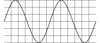### The period of the wave is 6.5 s. Don't make the mistake of thinking the period is measured from one midpoint to the next; that value (3.25 s in this case) is half the period.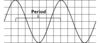5

## Which waveform is oscillating more rapidly, if the period of waveform 1 is twice that of waveform 2?

### Waveform 2 is oscillating more rapidly. The period is the time for the waveform to complete one full oscillation. The larger the period, the more time it takes for an oscillation to complete, the slower the oscillation.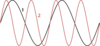6

7

8

## What is the frequency of the waveform shown below, if each horizontal division represents 1 second?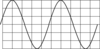### The waveform's frequency is 0.2 Hz.The period of the waveform is 4.5 s, and the frequency f = 1/T, or 1/4.5 s-1.9

10

## the phase of a waveform

### The phase of a waveform is the offset of the waveform relative to its origin (zero value). Although phase can hold any value, on the AP Physics exam the only commonly-tested values are integer amounts of quarter-wavelengths.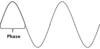11

## What is the phase difference between two waveforms?

### The phase difference between two waveforms is the value of the phase of the second waveform at the origin of the first waveform. Ex: The picture below represents a phase difference of one-half of a wave. Notice that point A is the origin of the red waveform, while the black waveform is halfway through a full oscillation.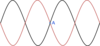12

## The two waveforms below differ by one-half of a wave. What is the calculated phase difference in: degrees? radians?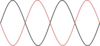13

## The two waveforms below differ by one-quarter of a wave. What is the calculated phase difference in: degrees? radians?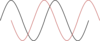14

## Hooke's Law for spring force

### Hooke's Law relates the extension of a spring to the force exerted by the spring. Fx = -kx Where: Fx = the force exerted by the spring in N k = the force constant of the spring in N/m x = the extension of the spring from equilibrium in m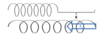15

16

17

## What is the shape of the graph for the motion of any simple harmonic motion system? (assume no frictional forces)

### All simple harmonic motion systems have a sinusoidal graph. Ex: In the below graph, displacement is graphed vs. time for a mass on a spring system.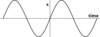18

## How would the graph for a mass on a spring look different if the mass begins to oscillate more quickly?

### The peaks of the graph will move closer together. Ex: In the graph below, the red line represents a system which is oscillating more rapidly.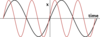19

## When is the point of maximum kinetic energy for a system oscillating with simple harmonic motion?

### The system has maximum kinetic energy when the object is at the equilibrium position. Kinetic energy is proportional to the square of the velocity, and velocity is the slope of the line tangent to the position curve, shown below. The slope maximizes as the line crosses through equilibrium, and is at a minimum (0) at the extreme positions.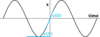20

21

22

23

24

25

26

27

28

29

30

31

32

## Transverse wave

### In a transverse wave, the particles oscillating move with displacement perpendicular to the direction of propagation.33

## What are some classic examples of transverse waves?### Transverse waves include: light waves (electromagnetic waves) string waves pond waves* stadium waves *Technically water waves also fall under the classification of "surface waves", but the AP Physics exam does not require that definition.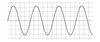34

## Longitudinal wave

### In a longitudinal wave, the particles oscillating move with displacement parallel to the direction of propagation.35

## What are some classic examples of longitudinal waves?

### Logitudinal waves include: sound waves stretched slinky waves (spring waves)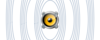36

## Calculate the period of the wave shown below:### The period is 5 seconds. A full wave cycle is any 360 degree segment and is usually easiest to see as peak-to-peak, or valley-to-valley. Notice that the wave valley hits exactly at 0, 5, 10, etc.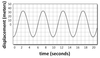37

## Calculate the frequency of the wave below:### The frequency is .2 Hz. Since frequency = 1/T, and the period is 5 seconds, this gives f = 1/5 = .2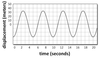38

## Calculate the amplitude of the wave below:### The amplitude is 17 meters. Amplitude is the greatest positive displacement from zero of a wave, measured at its peak.39

## Calculate the wavelength of the wave below:### The wavelength is 12.5 meters. A full wave cycle is any peak-to-peak, or valley-to-valley distance. Notice that the wave valley hits exactly at 0 and 25, but this requires two oscillations to cover this distance. Hence, wavelength is 25/2 = 12.5 for just one.40

## What is the speed of the wave below, if its wavelength is exactly 5 meters?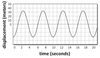### 1 m/s wave speed = λ * f = 5 (1/5) = 141

42

43

44

45

46

47

48

## standing wave

### A standing wave is one that exists at a fixed length in a given medium. An example of this is a guitar string that is stationary at both ends, but can have waves propogate between the two ends. Below is a guitar string vibrating at the 4th harmonic.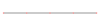49

## Nodes

### A node is the point on a standing wave that experiences zero displacement. Nodes remain fixed in position. In the image below, the black dots are the nodes.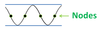50

## What condition must be met, in order for a node to exist at the end of a standing wave?

### For a node to exist at the end of the standing wave, that end must be fixed in position.  A guitar string tied down on both ends has nodes at both ends. An organ pipe with one end closed has a node at the closed end only.51

## antinode

### An antinode is the set of positions on a standing wave that experience the greatest displacement. A wave will fluctuate between antinode positions. In the image below, the black dots are the antinodes.52

## What condition must be met, in order for an antinode to exist at the end of a standing wave?

### For an antinode to exist at the end of the standing wave, that end must be open (not fixed). The pipe on a wind chime with both ends open has antinodes at both ends. An organ pipe with one end open has an antinode at the open end only.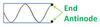53

54

55

56

57

58

59

60

61

## How does the sound wave physically propogate, as described by looking at specific particles of the medium?

### Particles pulse outward from some initiation point, towards their neighbors, creating a high density region. That high density wave-front continues outward, while the original particles settle back into the now much lower density region that they initially occupied. This high-and-then-low density pulse pair is a sound wave, and can exist in any medium.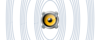62

63

64

65

66

67

68

69

70

71

72

73

74

75

76

77

78

79

80

81

82

83

84

85

86

## What physical concept, relating to sound, is the arrow pointing to?### A node. Nodes are the part of a standing wave that remains fixed in position.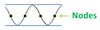87

## What physical concept, relating to sound, are the arrows pointing to?### Antinodes. Antinodes are the positions of greatest displacement for a standing wave.88

## Describe the specific standing wave that can exist in a pipe with both ends open.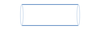### A pipe with both ends open must have antinodes at both ends, and at least one node between them. The wave below is the 4th harmonic, since it has 4 nodes.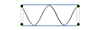89

## Describe the specific standing wave that can exist in a pipe with only one end open and the other closed.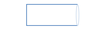### A pipe with only one end open must have an antinode at that end, and a node at the closed end. The wave below is the 7th harmonic, since it must pass through 7 nodes (3 in, 1 on end, 3 back out).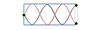90

## Describe the specific standing wave that can exist on a string with both ends fixed in place.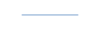### A string with both ends fixed must have nodes at both ends, and at least one antinode between them. The wave below is the 3rd harmonic, since it has 3 antinodes.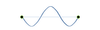91

92

93

## What will the fourth harmonic look like for a wind chime pipe that has both ends open?

### Antinodes at both ends, and four nodes (with corresponding antinodes) between them.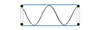94

## What will the fifth harmonic look like for an organ pipe that has only one end open?

### An antinode at the open end and a node at the closed end, and two nodes (with corresponding antinodes) between them. There are 5 nodes total that the wave passes through (2 in, 1 on the end, 2 back out).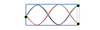95

## What will the third harmonic look like for a guitar string that has both ends fixed in place?

### Nodes at both ends, and three antinodes (with corresponding nodes) between them.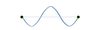96

97